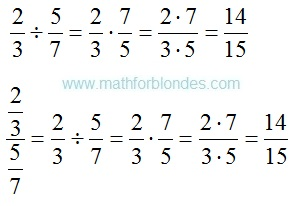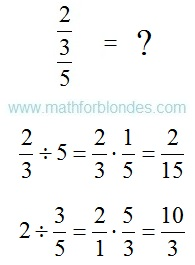## Pages

Showing posts with label fraction. Show all posts
Showing posts with label fraction. Show all posts

## 2.06.2017

### How to divide fraction into fraction?

When performing calculations often there is a question: "How to divide fraction into fraction?". Mathematicians rules tell so:

To divide a number by a fraction, multiply that number by the reciprocal of that fraction.How to divide fraction into fraction?
Transform fraction to division if in numerator and a denominator there are fractions. Be attentive! The numerator and denominator of fraction need to be known. The result depends on it.The numerator and denominator

As fraction to divide into fraction. Formula of division of fractions.Formula of division of fractions
You believe that in mathematician there is a division? The fraction cannot be divided into fraction. They can only be multipled.

## 4.09.2016

### The equation a fraction

What if the equation of lead shot, and X is hidden in the denominator? We need to get rid of the denominator. What will happen with X? Do not worry. If you do everything correctly, he from the denominator goes into the numerator. Here you can recommend two ways to solve the equation.

The first method is to bring the expression to normal proportions. Then we use the proportion of the property - the multiplication of extreme terms in proportion equal to the multiplication of the average proportion of members. Denominator magically disappear. We get a simple equation, which can be easily solved. Consider an example.The equation a fraction

The number did not work nicely. Make checks and look at the result. Substitute the original expression instead of X its value.Checking equation

The audit showed that the equation is solved correctly.

The second method is to bring the expression to a common denominator. The denominator should be common to the left and right sides of the equation. In this case, we in good conscience can throw the denominator in the trash. We see an example of reduction to a common denominator.The equation a fraction
The result was the same as in the first case.

## 3.04.2016

### What time is it now?

Q: From the beginning of the day it took 3/4 days. What time is it now?

The day has 24 hours. Multiply the fraction by the number of hours in the day.

24 * 3/4 = 18 (hours)

From the beginning of the day it took 18 hours. Now we can calculate the time.

18:00 - 12:00 am = 6:00 pm

A: Now 18:00 or 6:00 pm.

## 10.11.2010

### Devidend of fraction as named?

The devidend of fraction is named numerator. A numerator is always written above a fractional line. It is needed nowhere to peep in search of numerator of fraction, type under a bed. A numerator of fraction is always on the most prominent position, from above fractional line, as a prince on a pea. As a numerator there can be numbers or mathematical expressions.

## 8.23.2010

### How is a devidend named, that under a line?

In a fraction under a line a divizor is written. He is named denominator. If you must find the denominator of fraction, it is needed to search him under a fractional line. Type, how under a bed to glance. All denominators are always hidden under a fractional line, as under a bed. As a denominator there can be both numbers and whole mathematical expressions, sometimes very large.

### Devidend of fraction as named?

The devidend of fraction is named numerator. A numerator is always written above a fractional line. It is needed nowhere to peep in search of numerator of fraction, type under a bed. A numerator of fraction is always on the most prominent position, from above fractional line, as a prince on a pea. As a numerator there can be numbers or mathematical expressions.